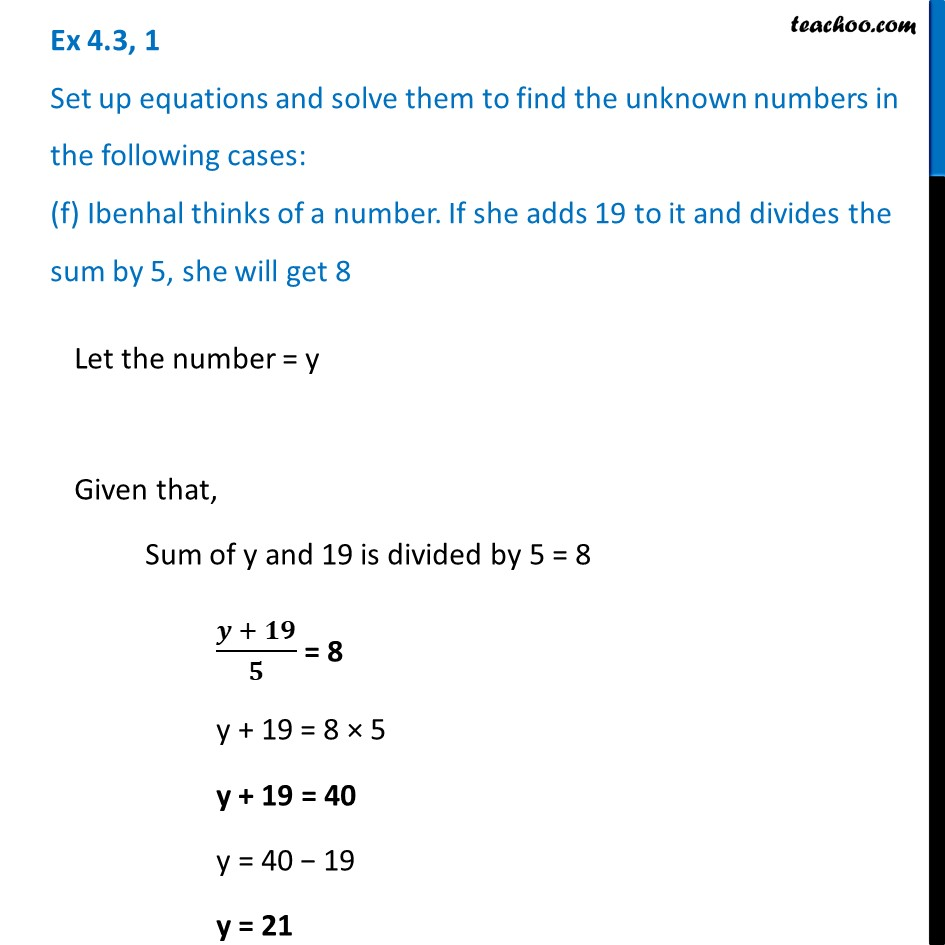Ex 4.3

Chapter 4 Class 7 Simple Equations
Serial order wiseLearn in your speed, with individual attention - Teachoo Maths 1-on-1 Class

### Transcript

Ex 4.3, 1 Set up equations and solve them to find the unknown numbers in the following cases: (f) Ibenhal thinks of a number. If she adds 19 to it and divides the sum by 5, she will get 8Let the number = y Given that, Sum of y and 19 is divided by 5 = 8 (𝒚 + 𝟏𝟗)/𝟓 = 8 y + 19 = 8 × 5 y + 19 = 40 y = 40 − 19 y = 21